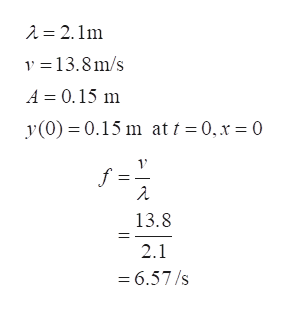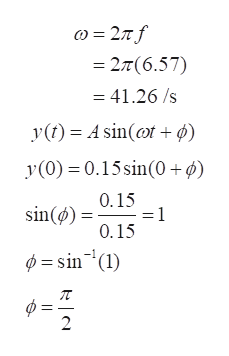# Write an expression for a transverse harmonic wave that has a wavelength of 2.1 m and propagates to the right with a speed of 13.8 m/s . The amplitude of the wave is 0.15 m , and its displacement at t=0 and x=0 is 0.15 m .

Question
16 views

Write an expression for a transverse harmonic wave that has a wavelength of 2.1 m and propagates to the right with a speed of 13.8 m/s . The amplitude of the wave is 0.15 m , and its displacement at t=0 and x=0 is 0.15 m .

check_circle

Step 1

Step 1

Harmonic wave is any wave which has repetitive frequency with respect to some initial wave. The transverse harmonic wave is the one which travels in the transverse direction.

The wave equation is given as

Step 2

Step 2

It is given that

Now,help_outlineImage Transcriptionclose2 = 2.1m v =13.8m/s A = 0.15 m y(0) = 0.15 m at t = 0,x = 0 f = 13.8 2.1 = 6.57/s fullscreen
Step 3

Step 3

Put these values in the main eq...help_outlineImage Transcriptionclose) = 27 f = 2¤(6.57) = 41.26 /s y(t) = A sin(@t + ø) y(0) = 0.15 sin(0 + ø) 0.15 sin(ø) = =D1 0.15 Ø = sin-'(1) Ø = fullscreen

### Want to see the full answer?

See Solution

#### Want to see this answer and more?

Solutions are written by subject experts who are available 24/7. Questions are typically answered within 1 hour.*

See Solution
*Response times may vary by subject and question.
Tagged in